# Femi Kuti & Positive Force

Always a big pleasure to hear Femi Kuti & Positive Force live. After many of his concerts I saw in Slovenia, Macedonia and New York, now again in Ljubljana at Kino Siska. It was hot and still positive. They are in action again.

•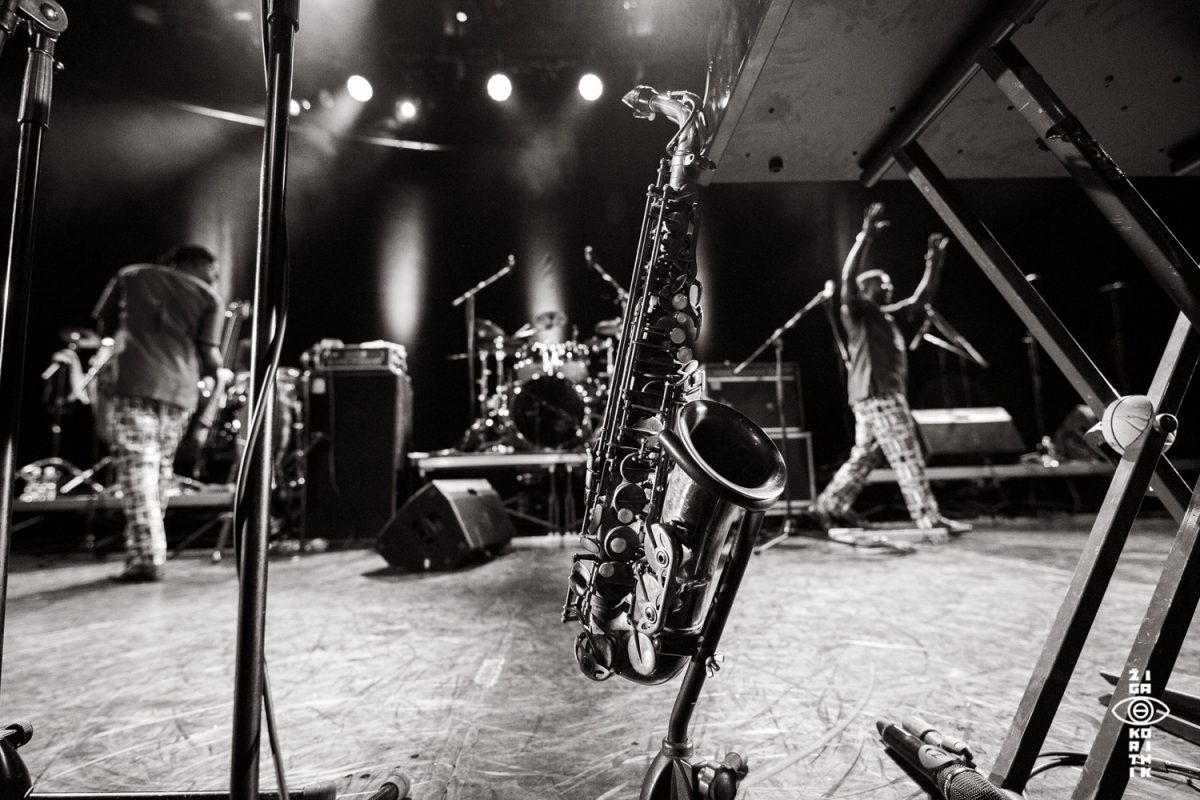Femi Kuti & Positive Force
•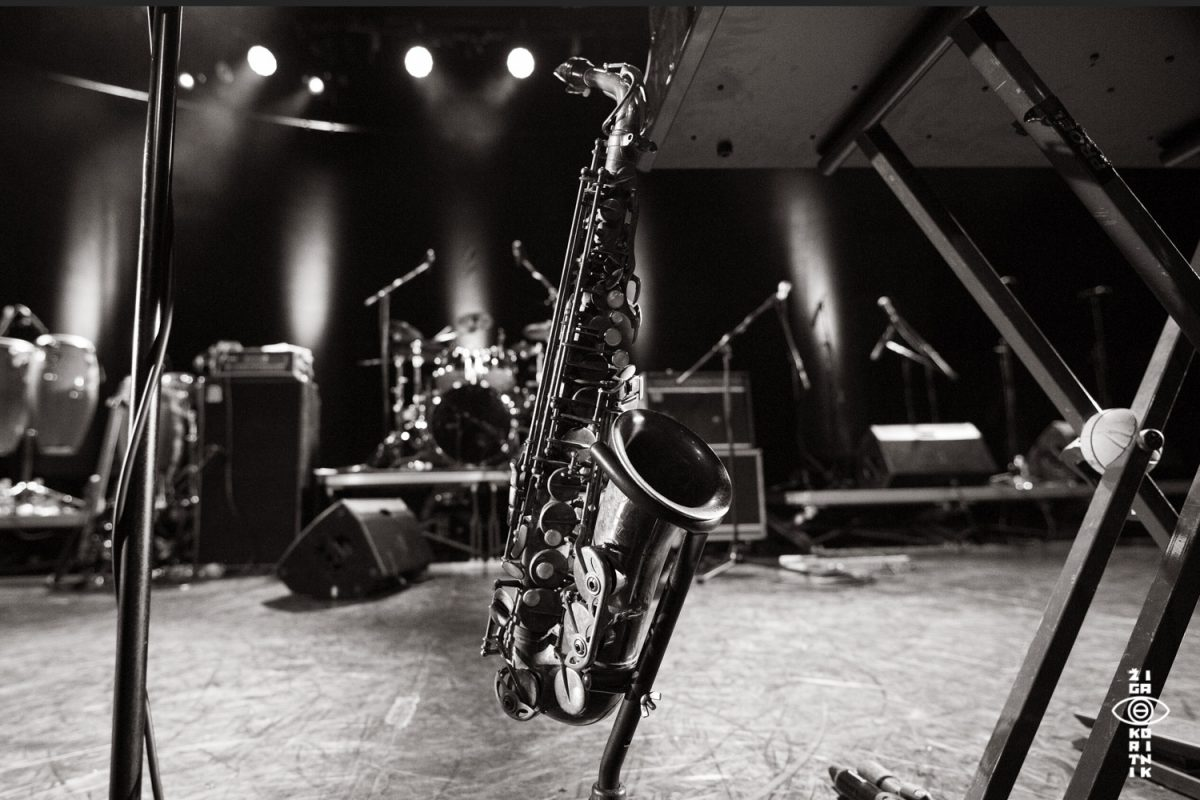FemiKuti_ByZigaKoritnik2018_2
•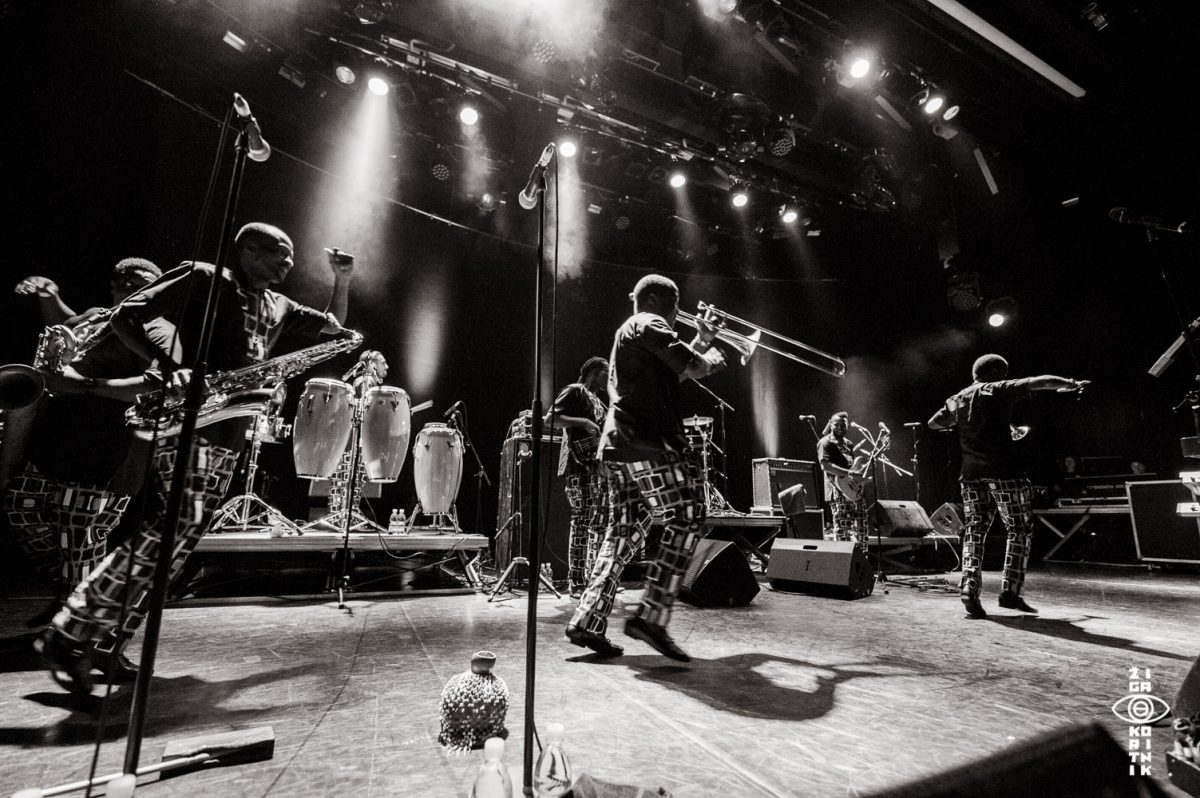Femi Kuti & Positive Force
•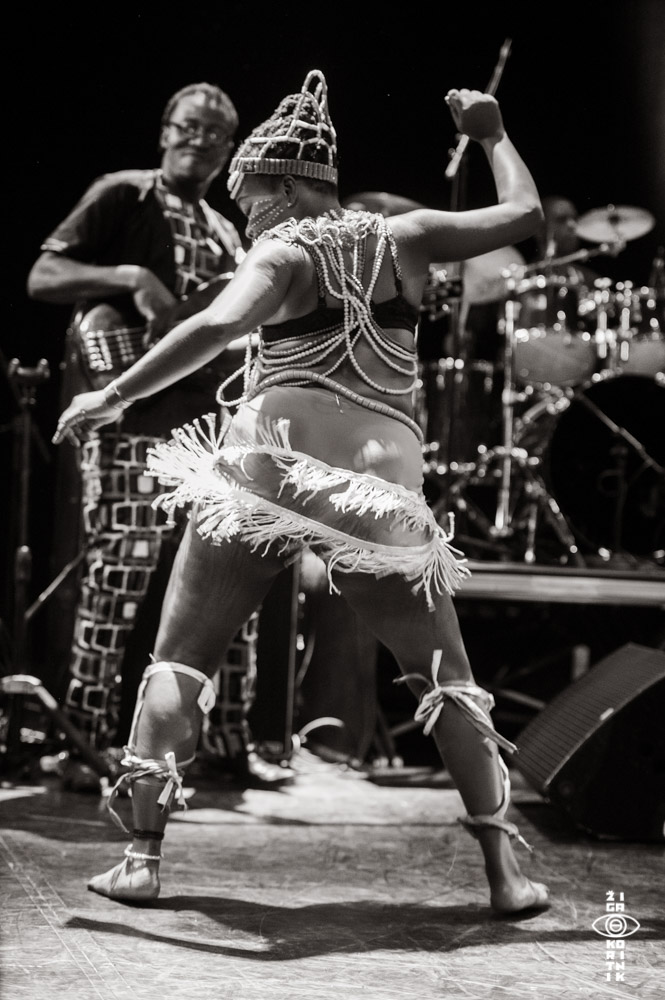Femi Kuti & Positive Force
•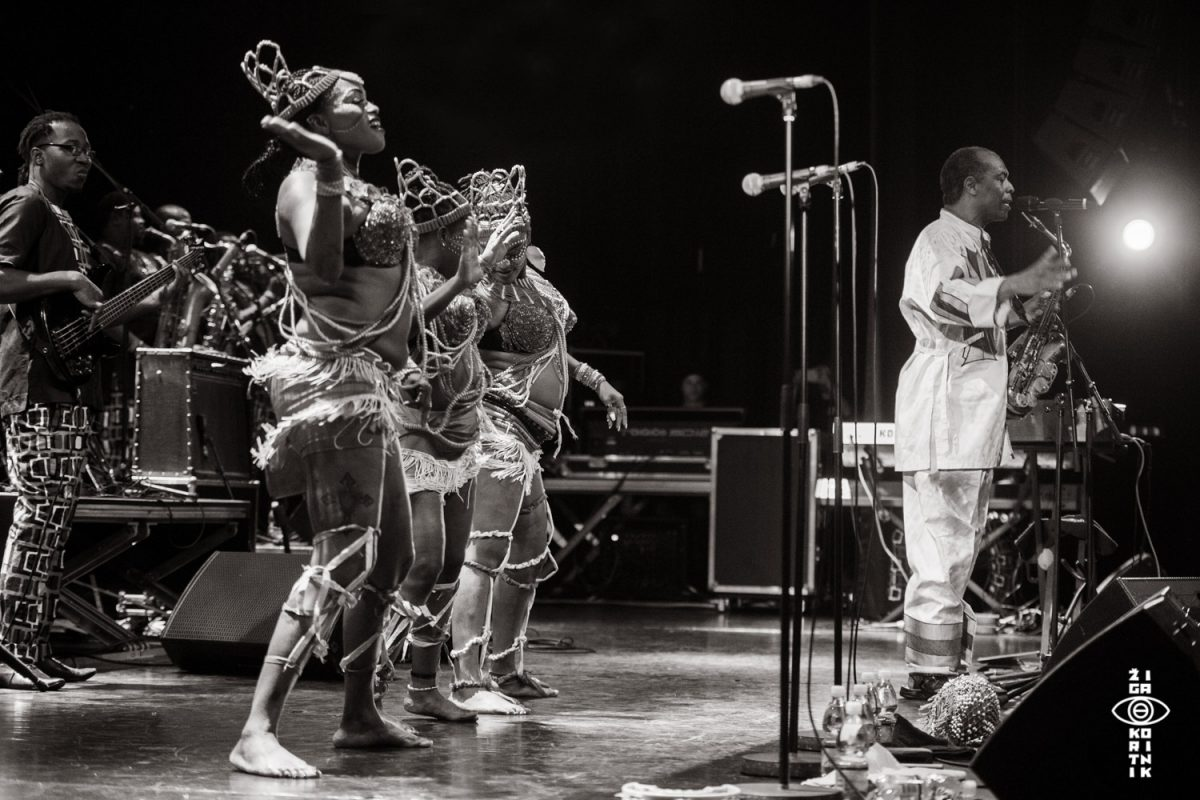Femi Kuti & Positive Force
•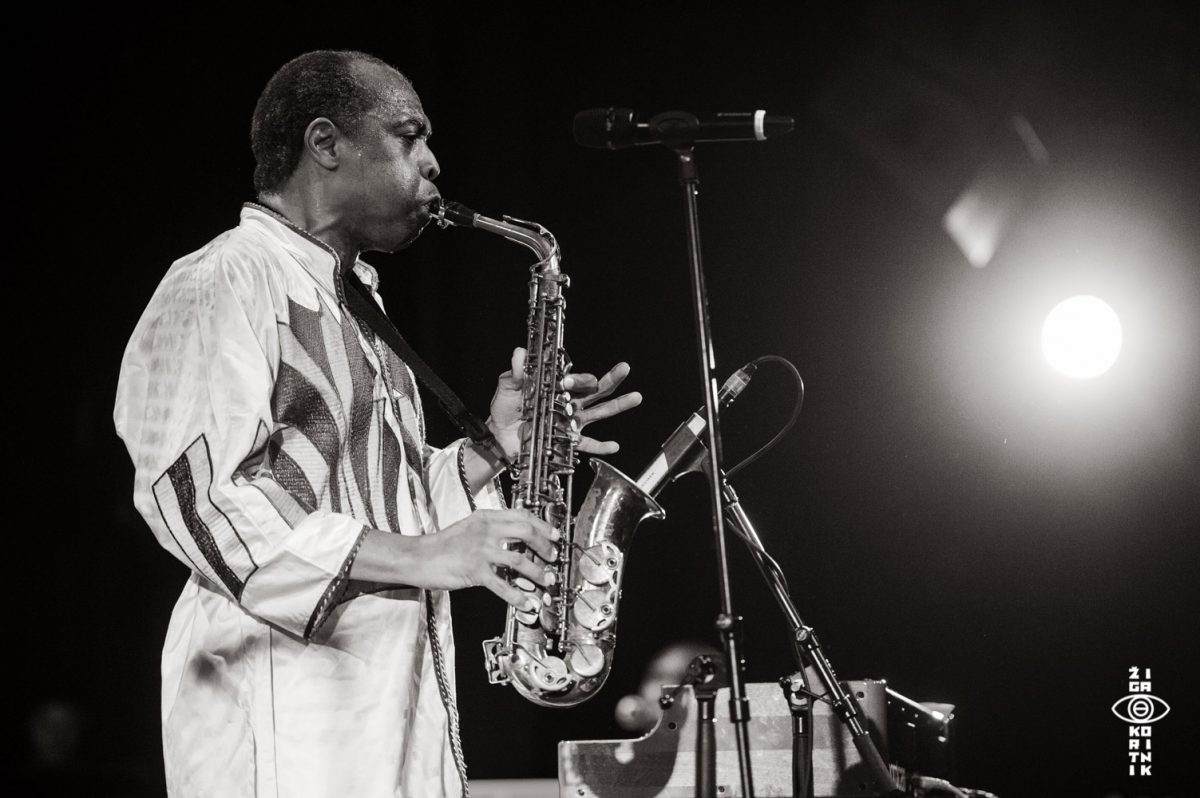Femi Kuti & Positive Force
•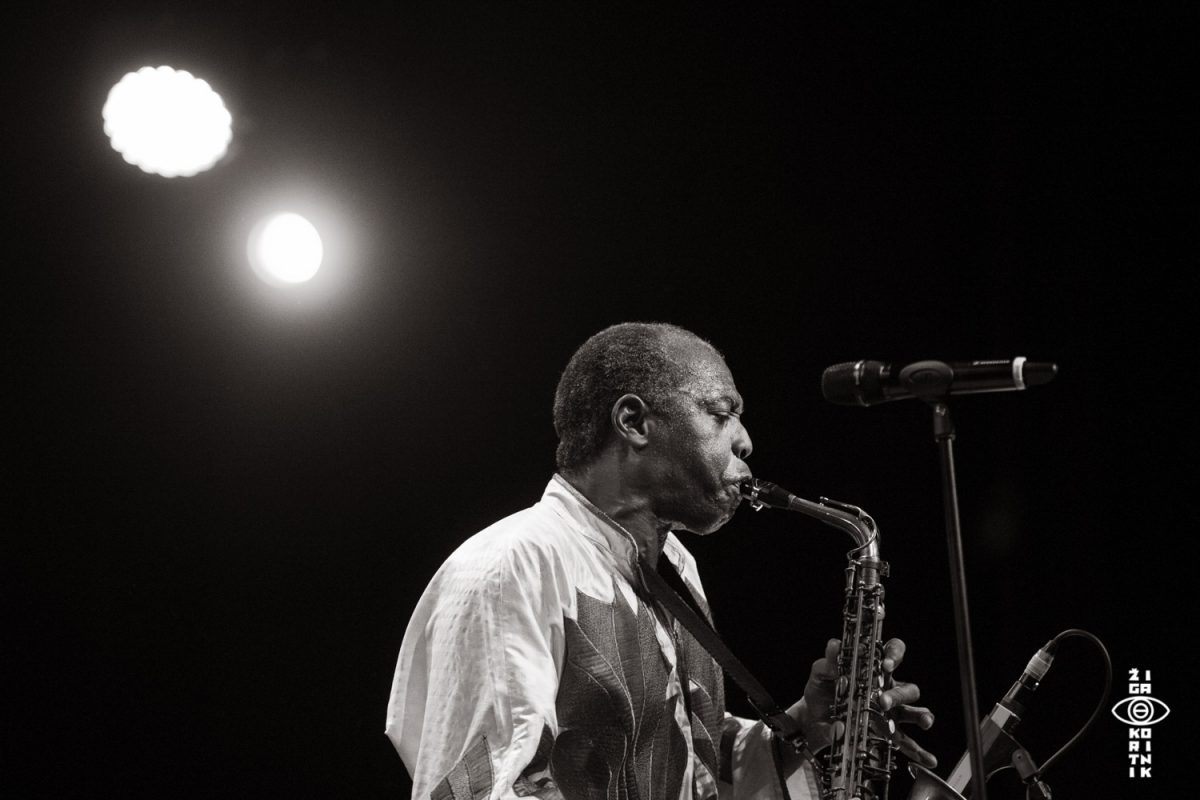Femi Kuti & Positive Force
•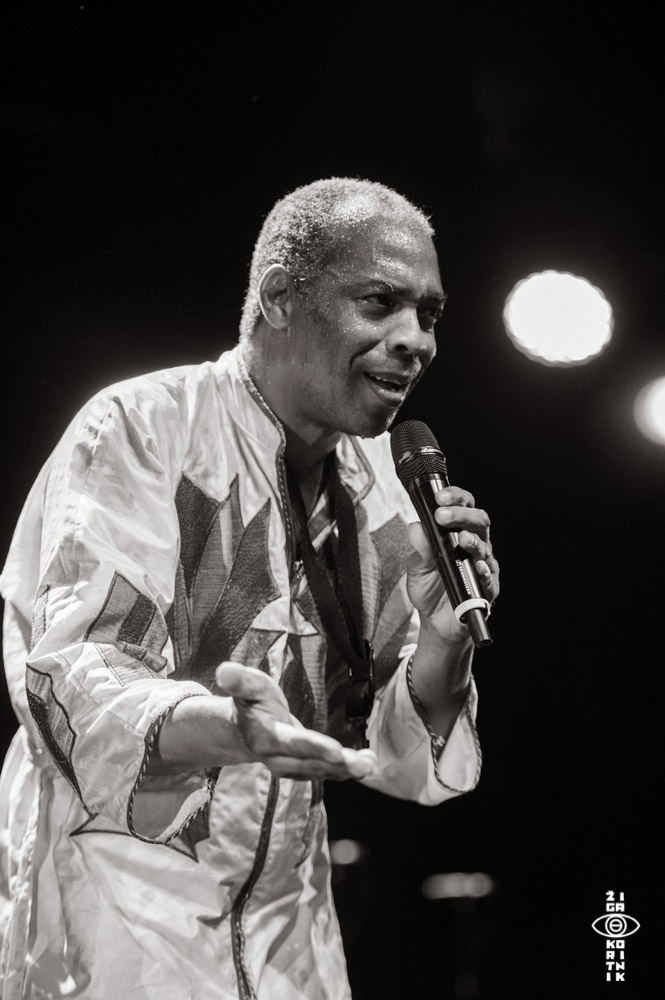Femi Kuti & Positive Force
•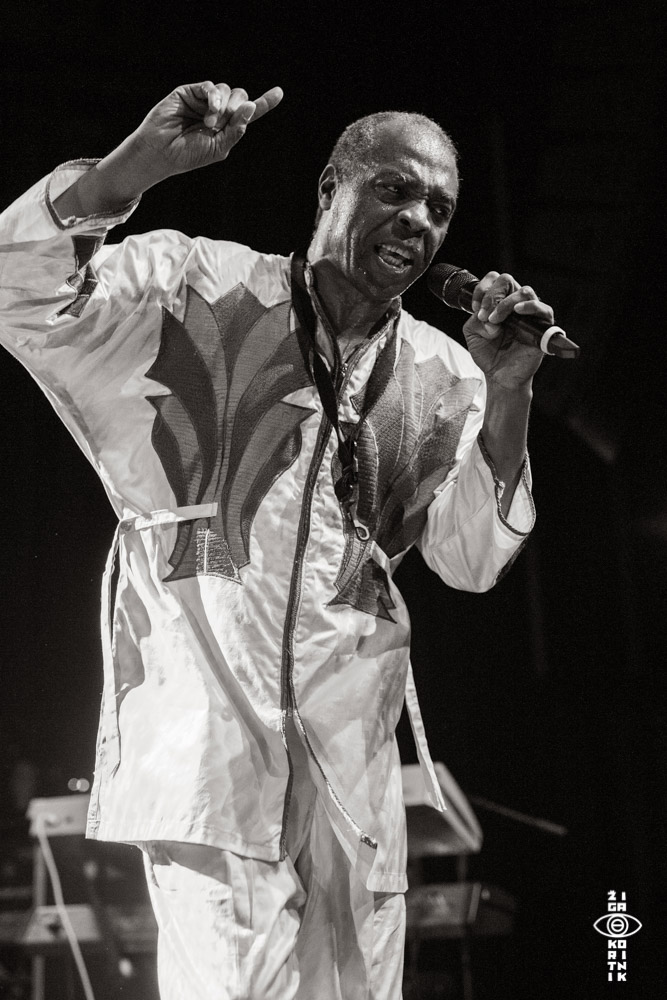Femi Kuti & Positive Force
•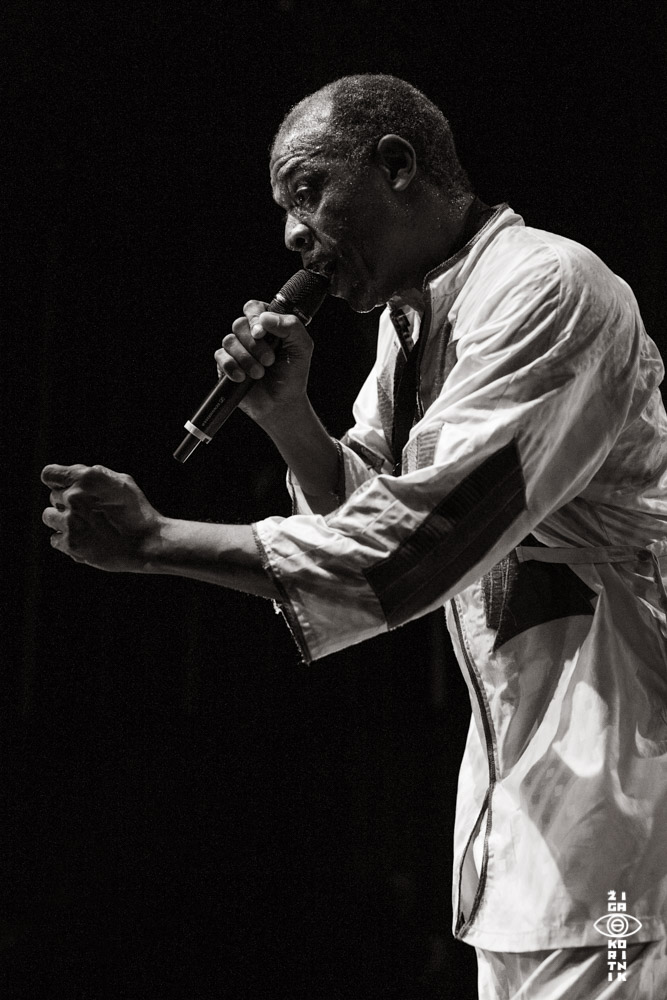Femi Kuti & Positive Force
•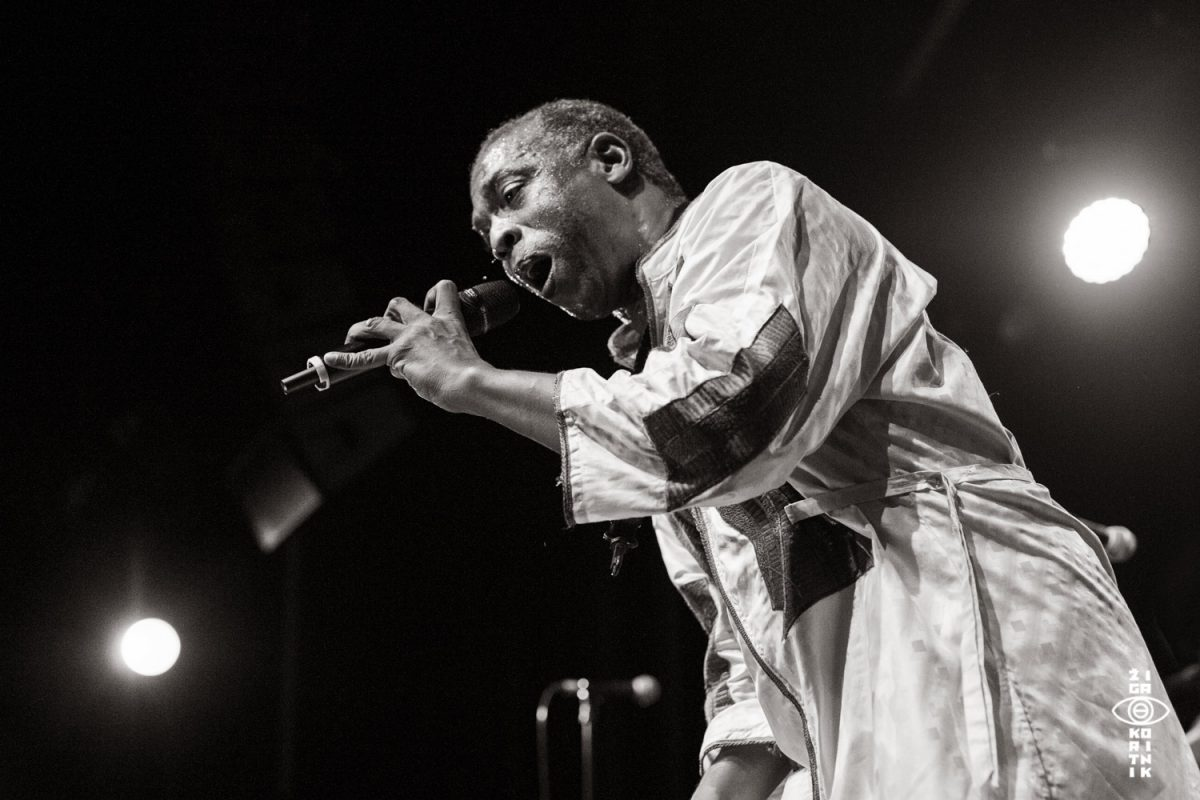Femi Kuti & Positive Force
•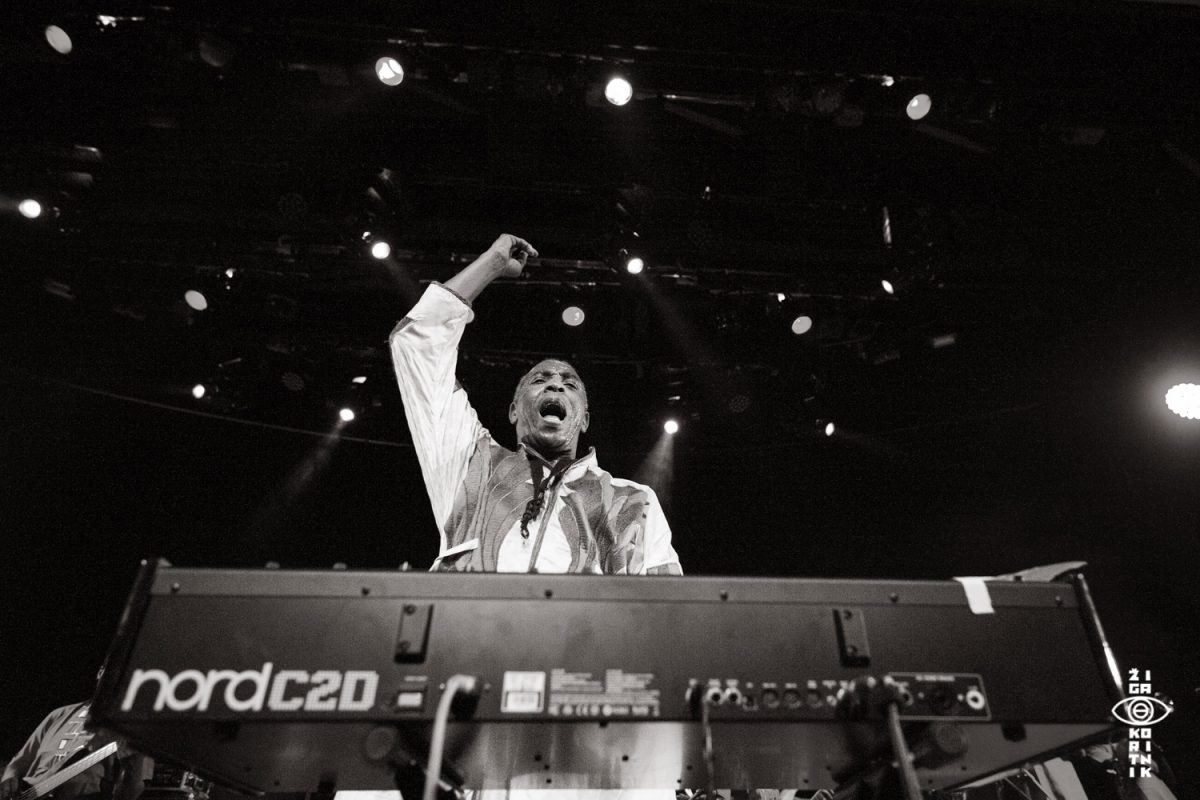Femi Kuti & Positive Force
•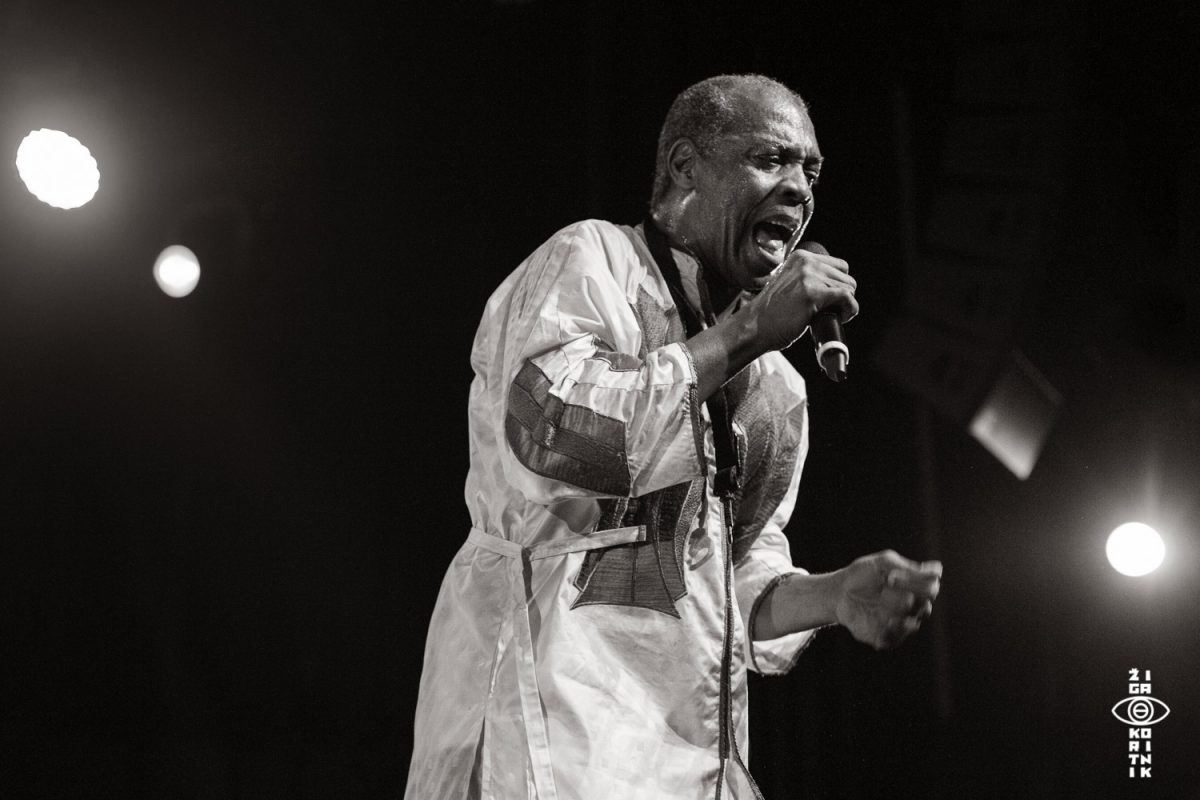Femi Kuti & Positive Force
•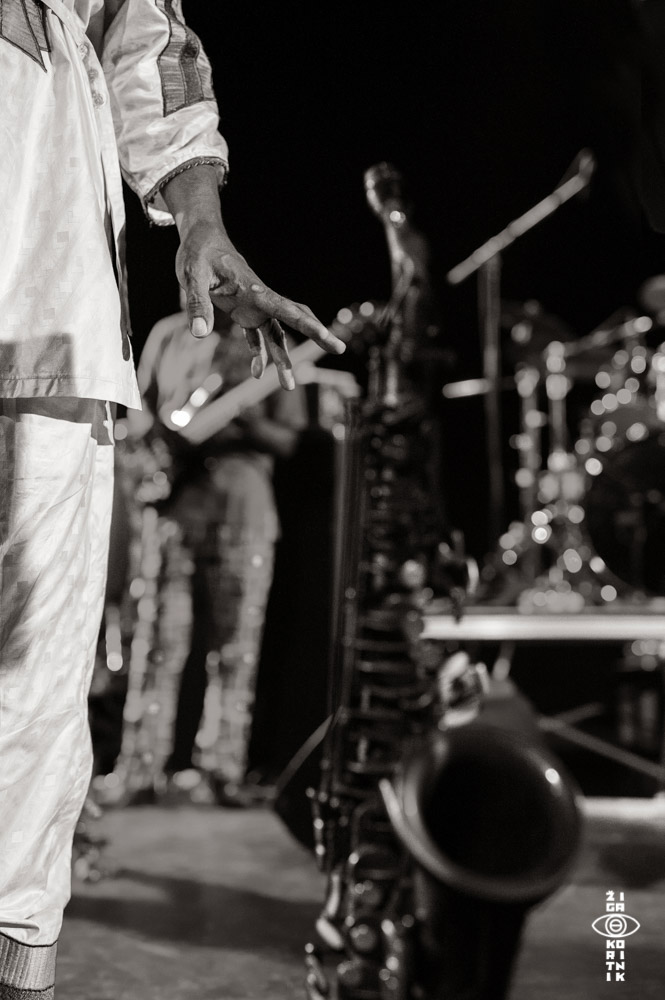Femi Kuti & Positive Force
•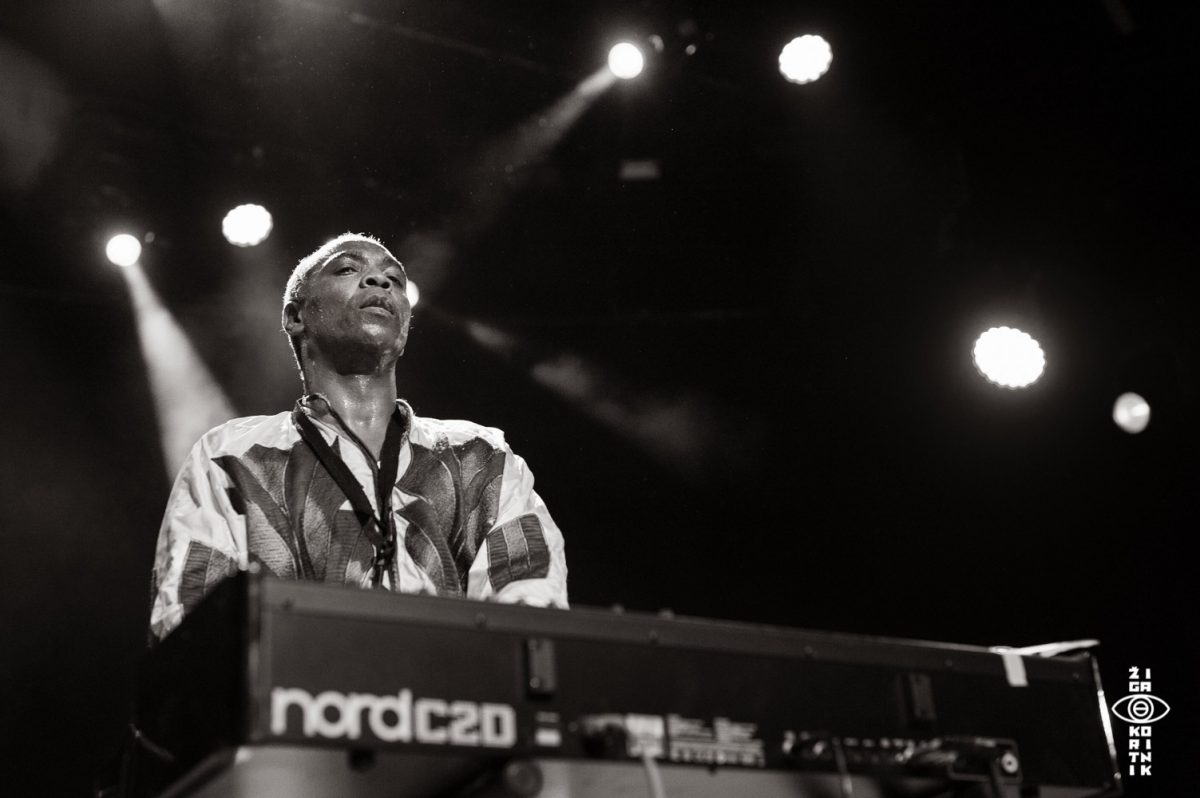Femi Kuti & Positive Force
•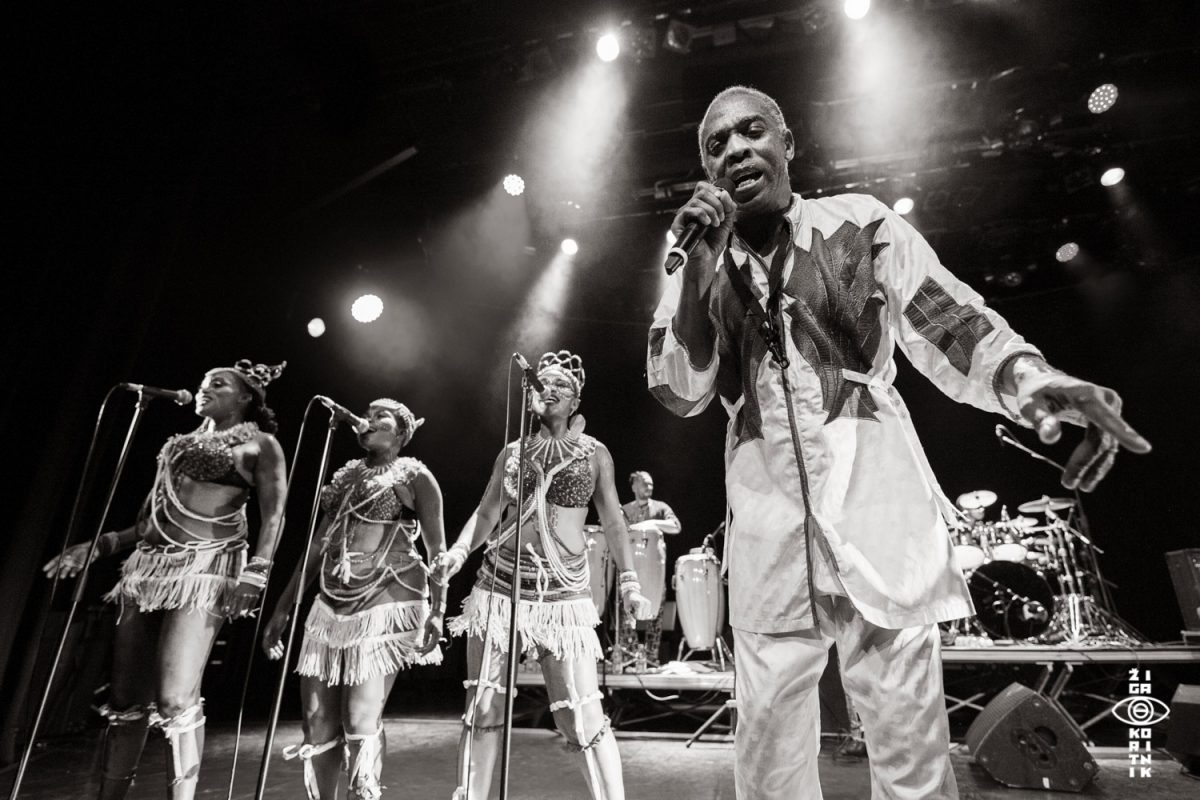Femi Kuti & Positive Force
•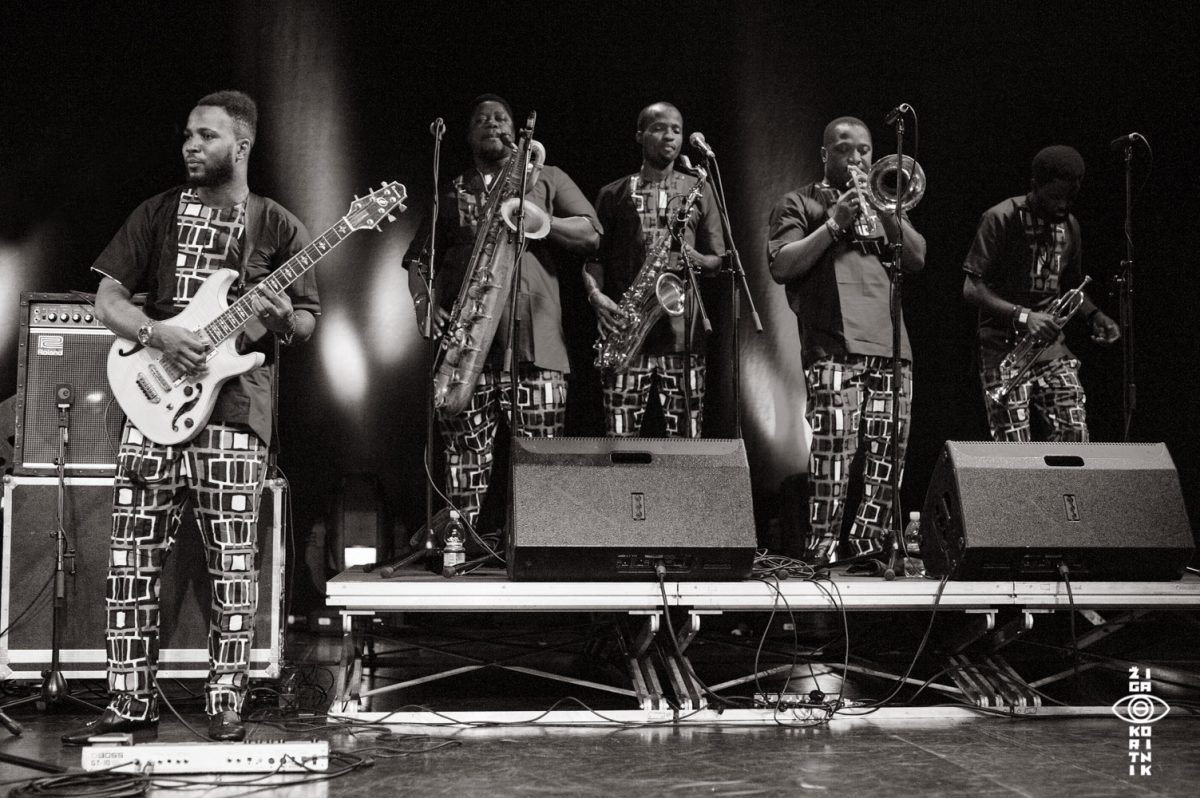Femi Kuti & Positive Force
•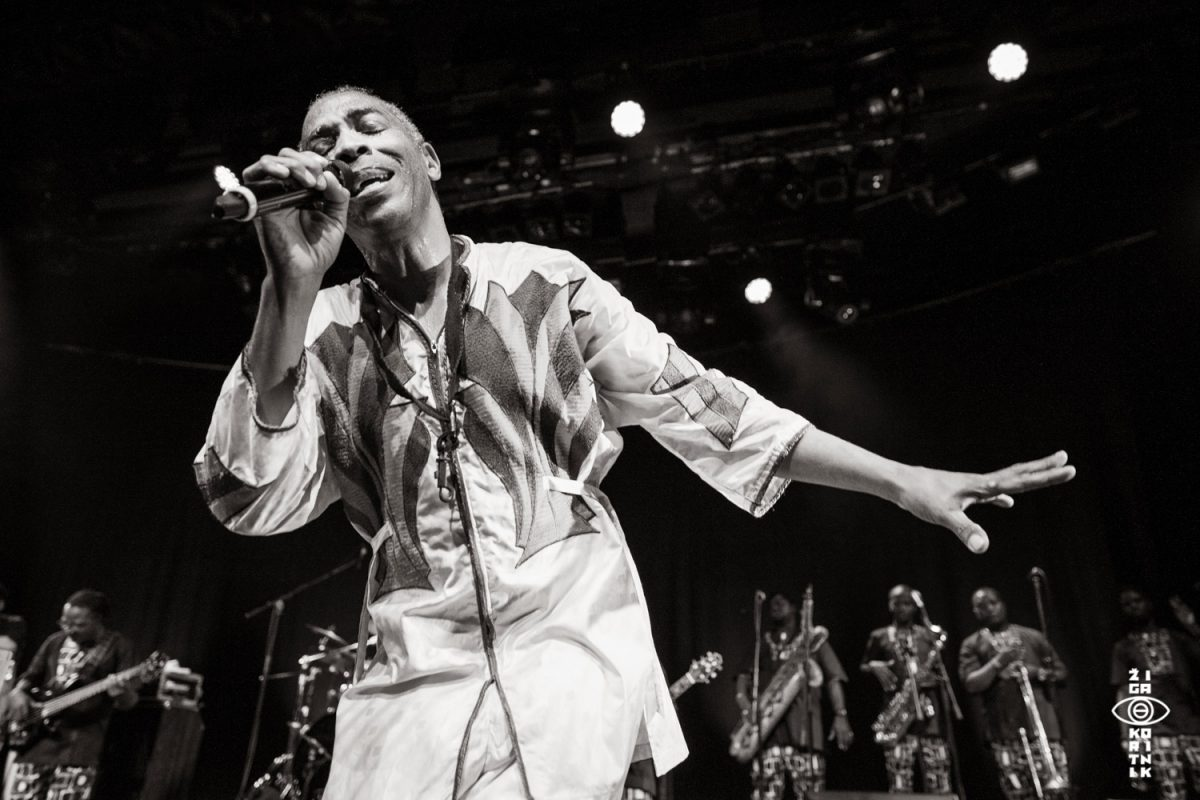Femi Kuti & Positive Force
•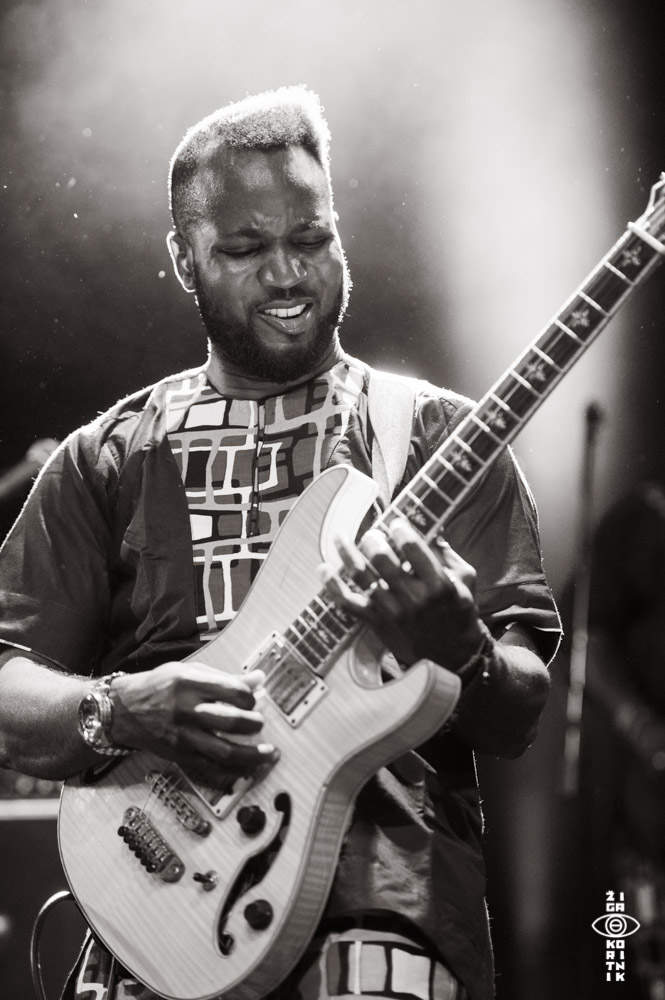Femi Kuti & Positive Force
•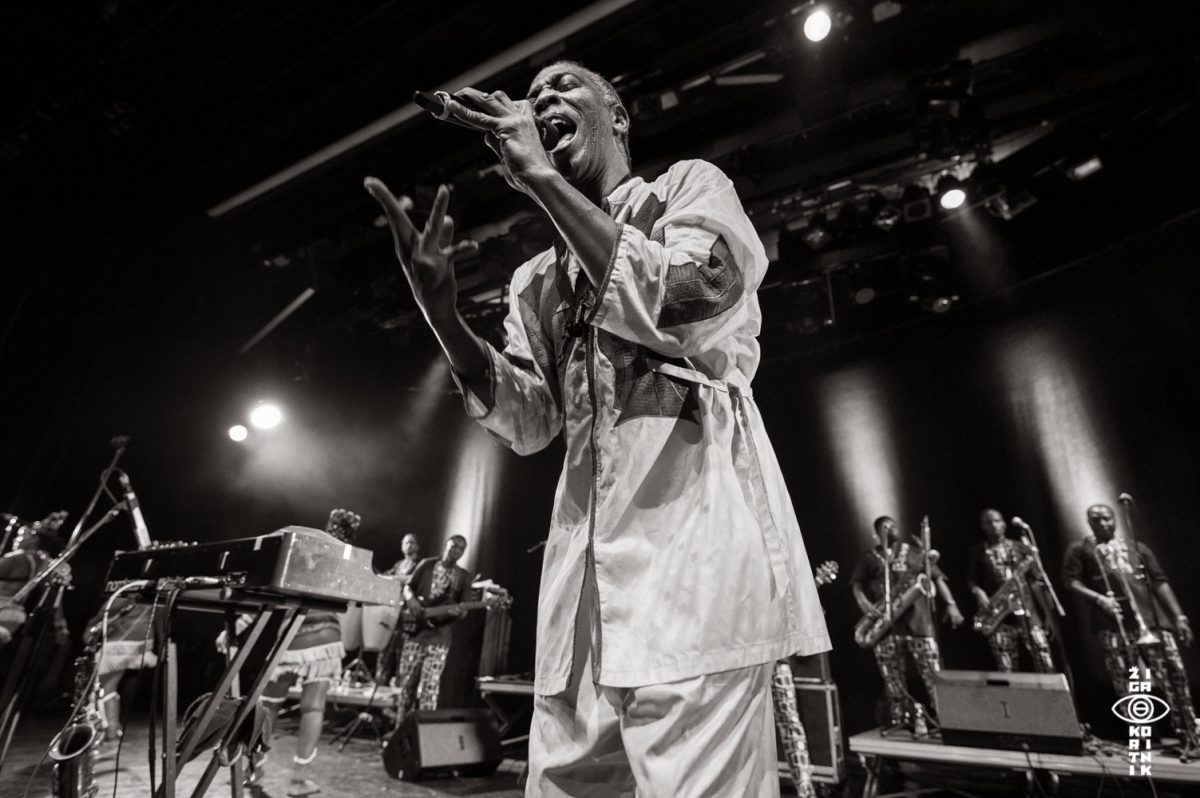Femi Kuti & Positive Force
•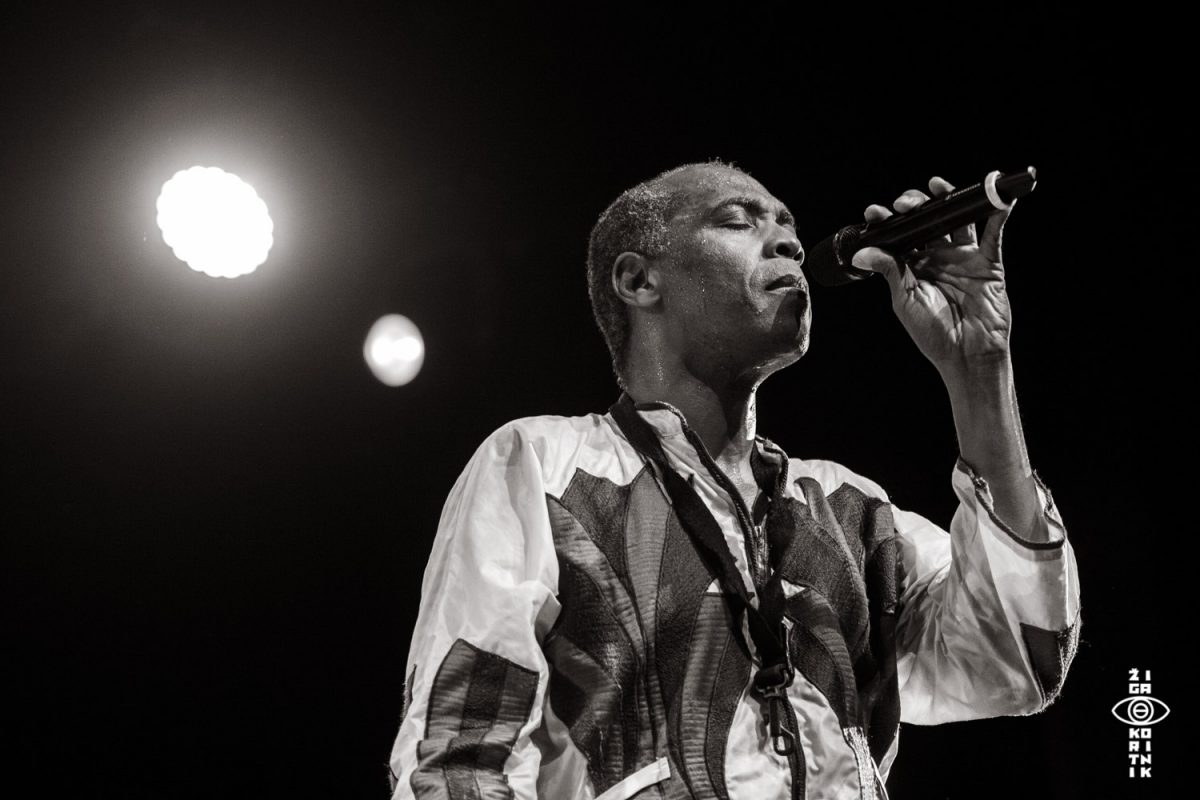Femi Kuti & Positive Force
•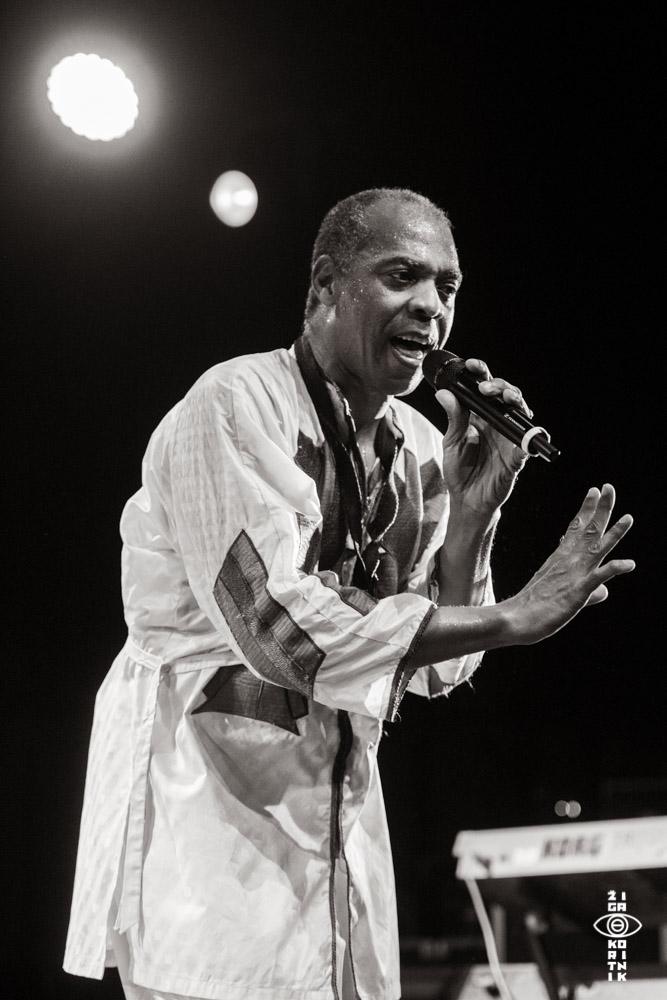Femi Kuti & Positive Force
•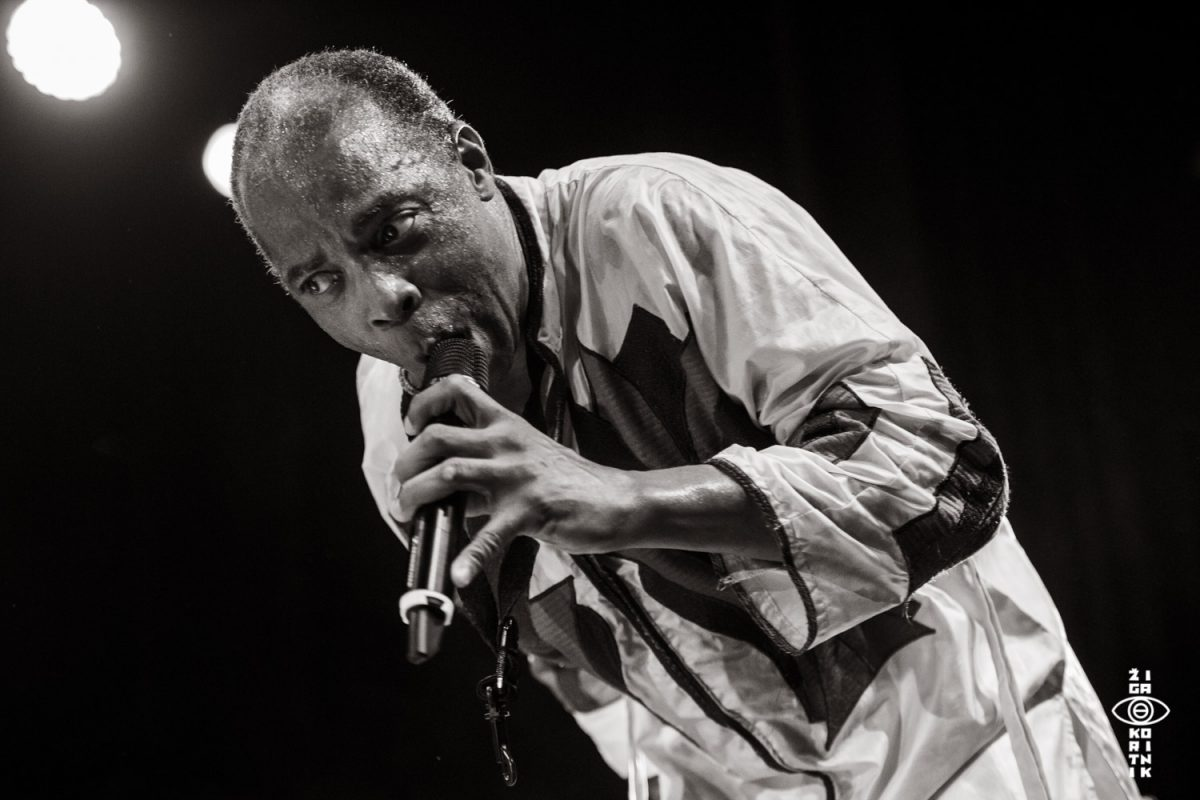Femi Kuti & Positive Force
•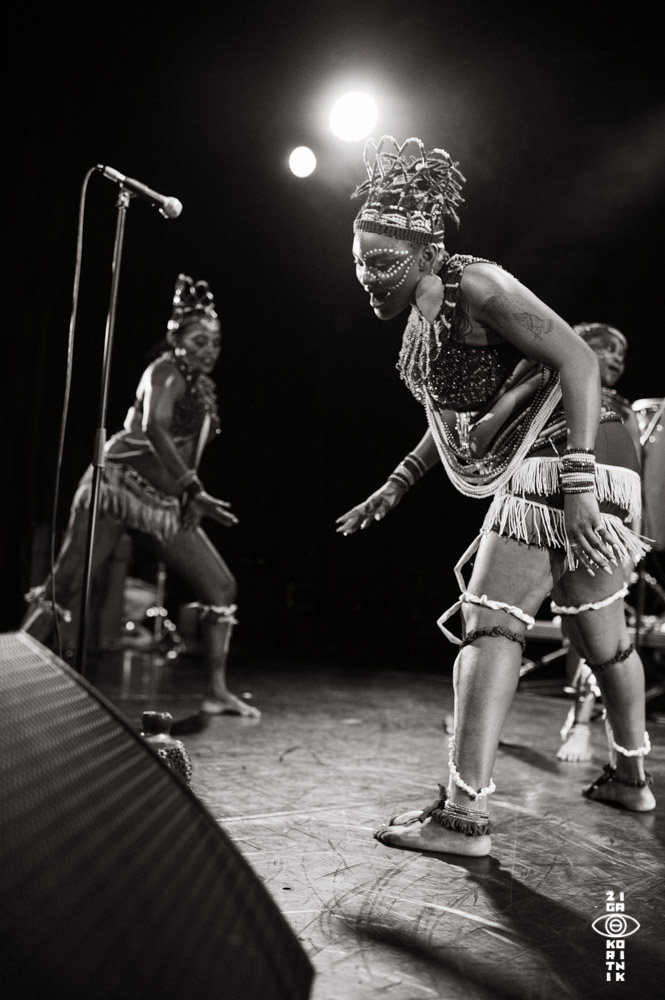Femi Kuti & Positive Force
•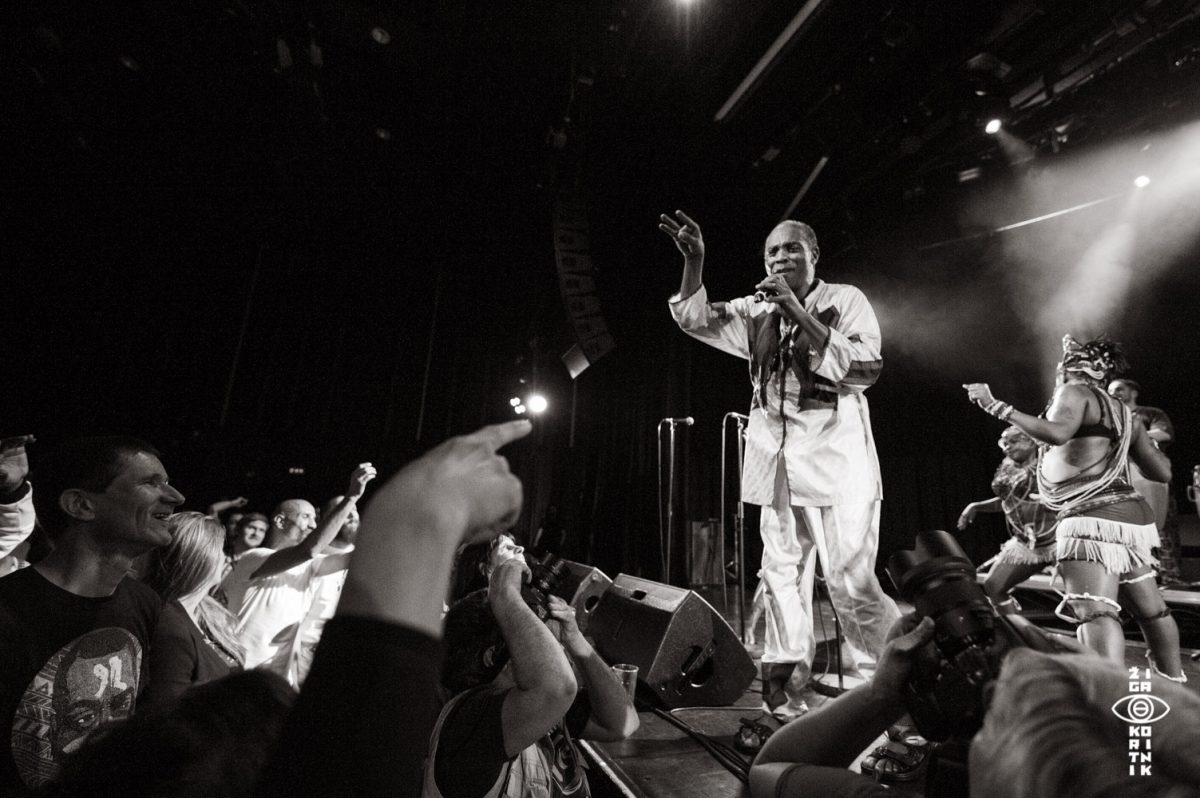Femi Kuti & Positive Force
•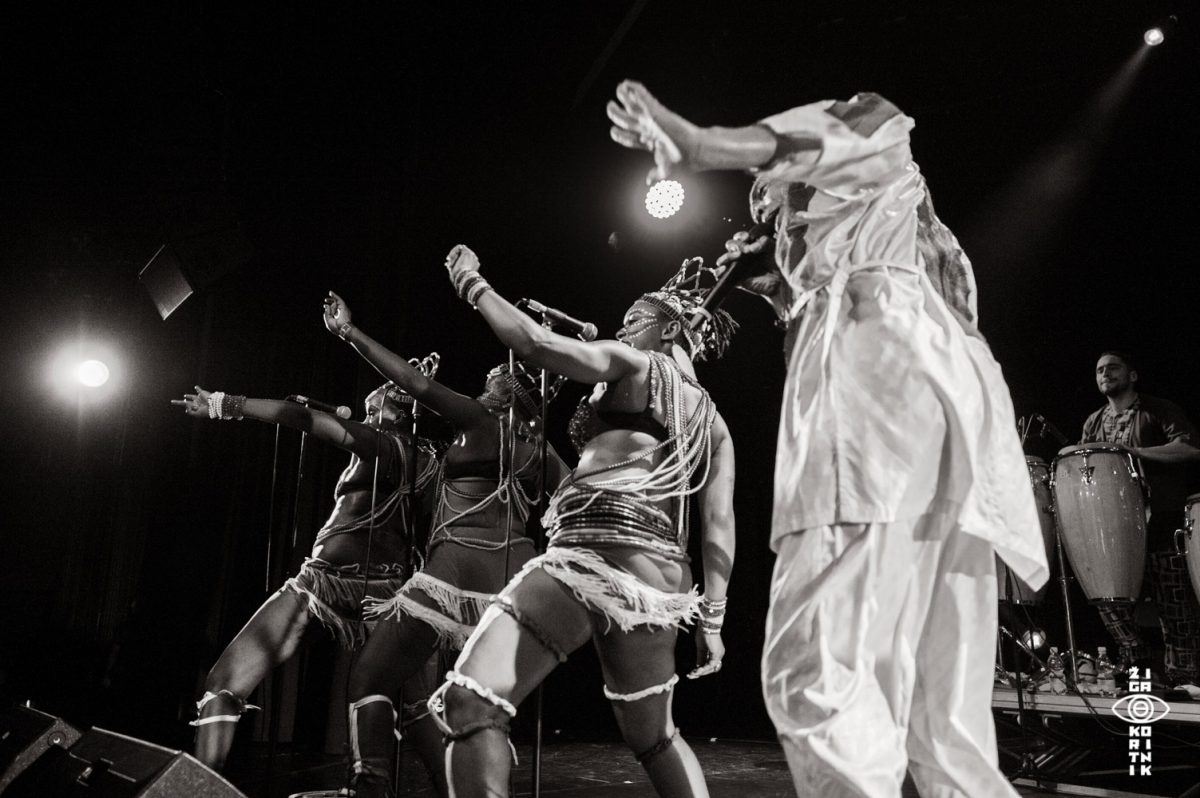Femi Kuti & Positive Force
•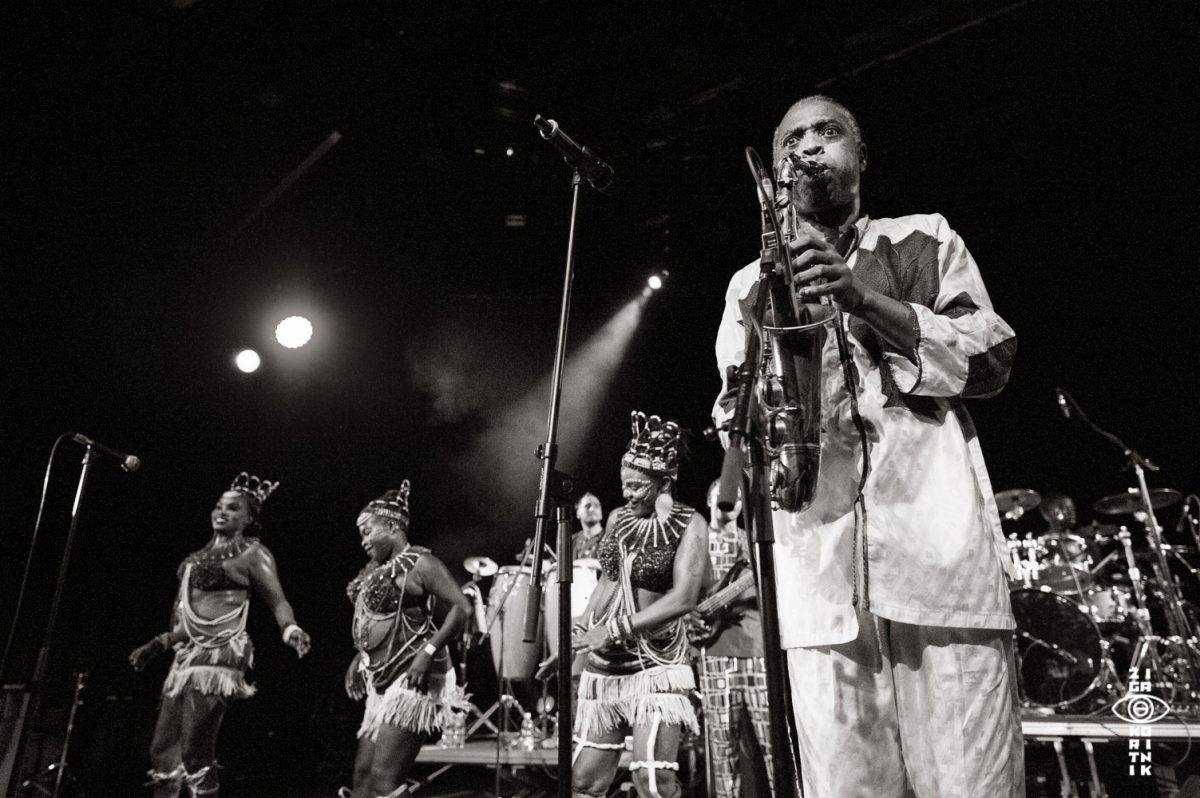Femi Kuti & Positive Force
•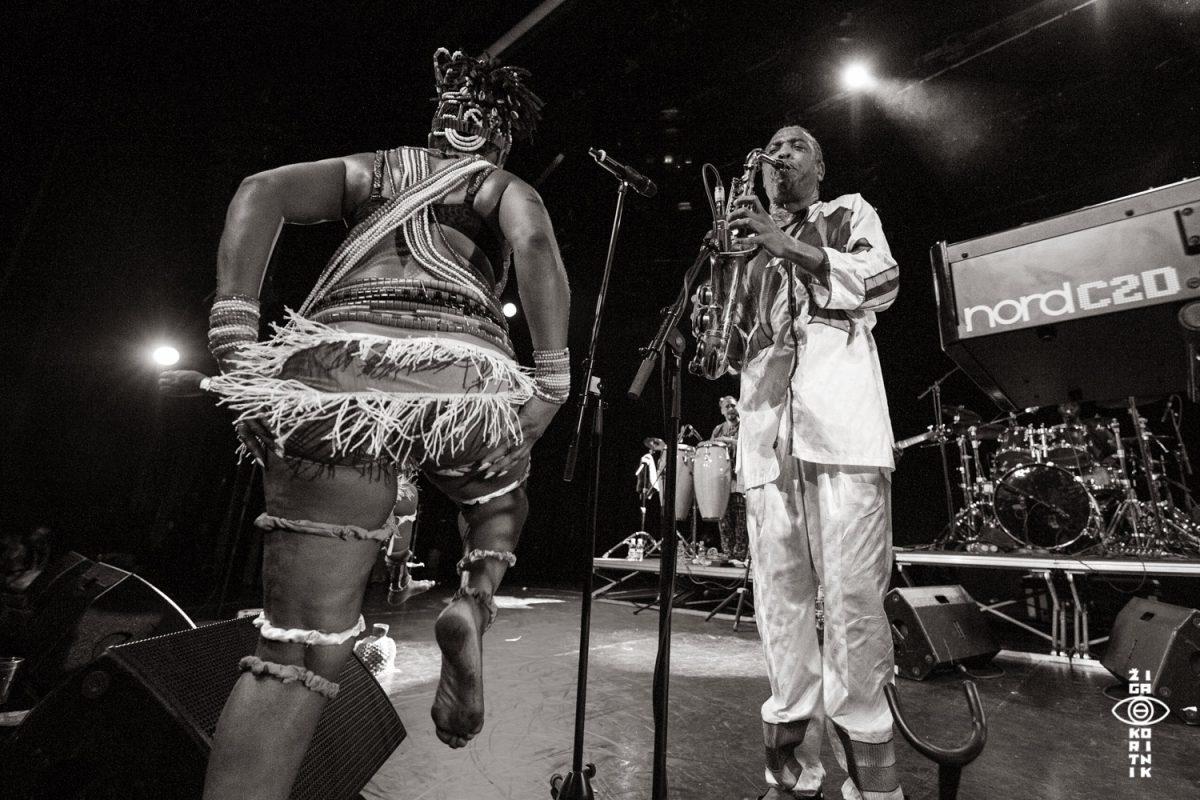Femi Kuti & Positive Force
•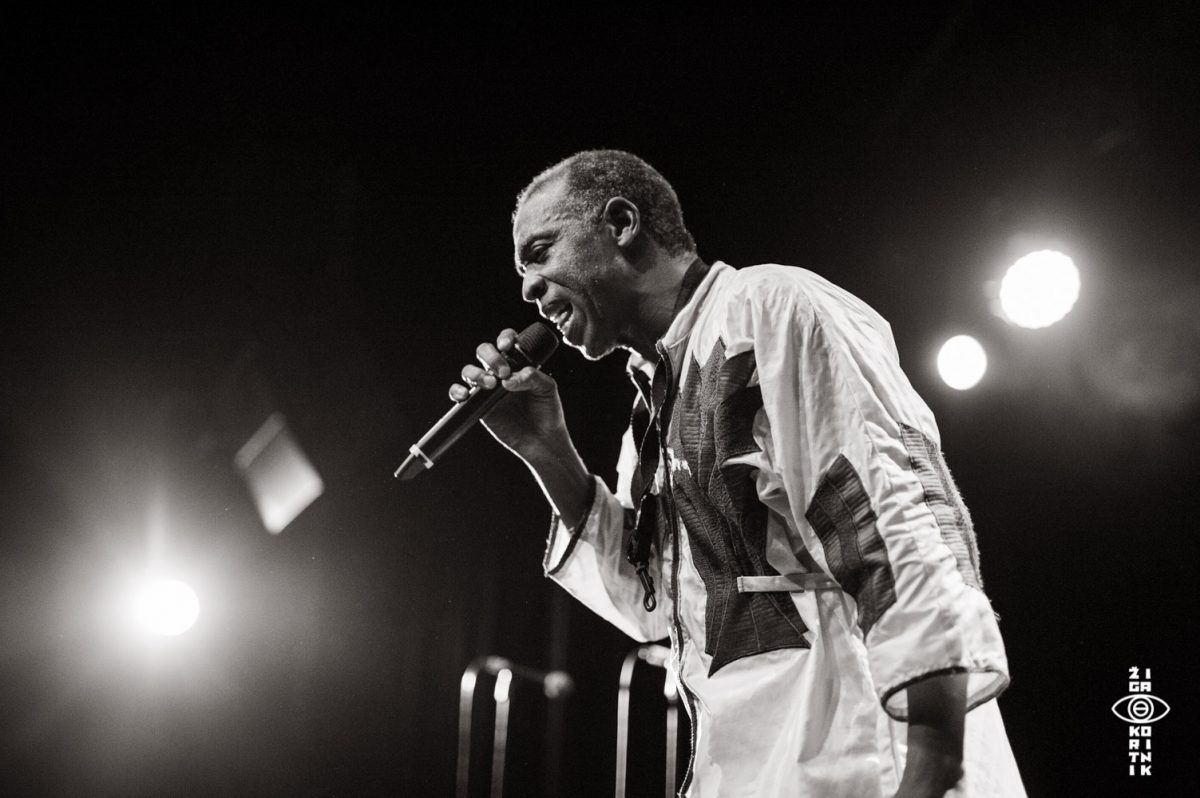Femi Kuti & Positive Force
•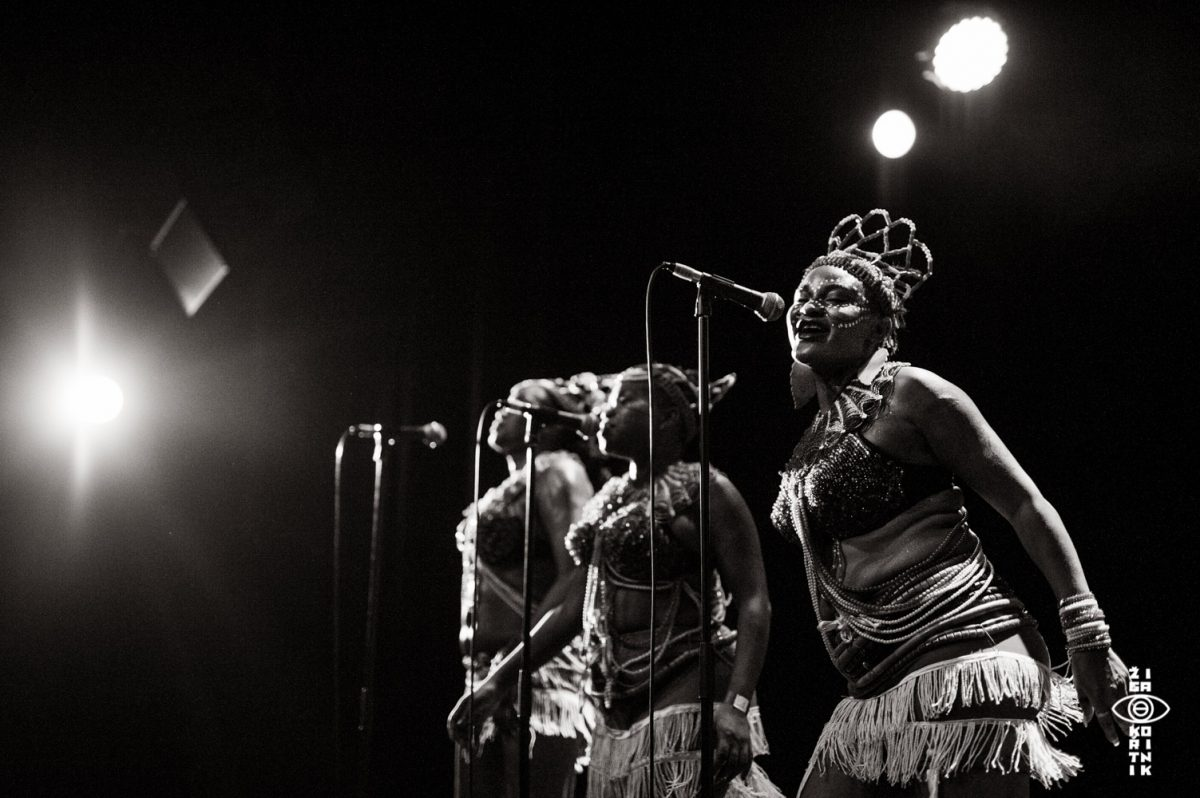Femi Kuti & Positive Force
•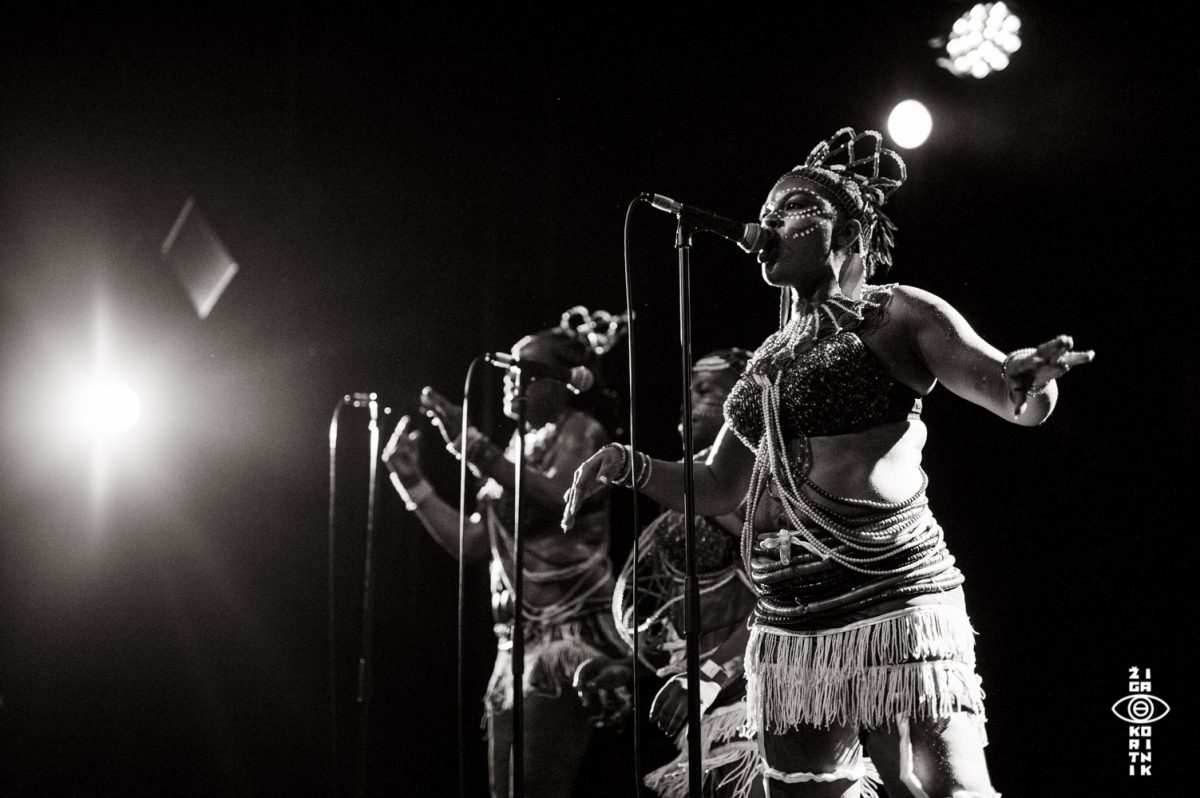Femi Kuti & Positive Force
•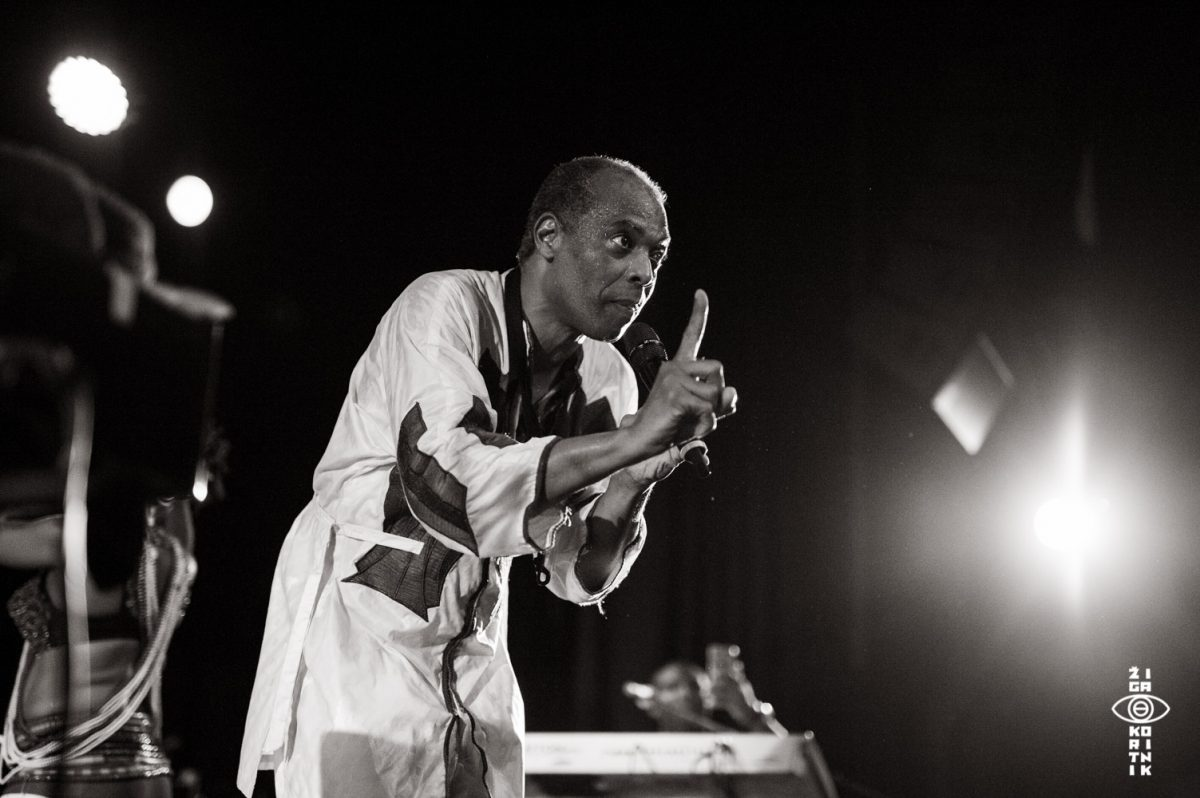Femi Kuti & Positive Force
•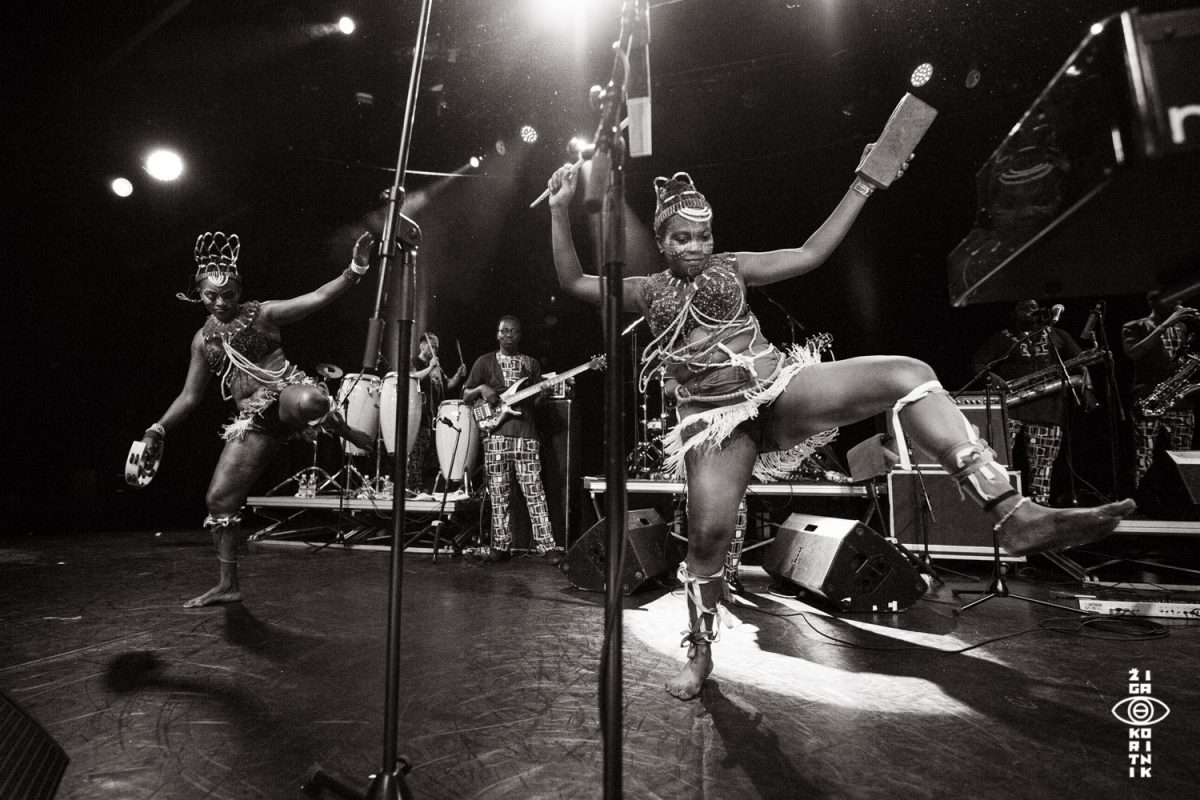Femi Kuti & Positive Force
•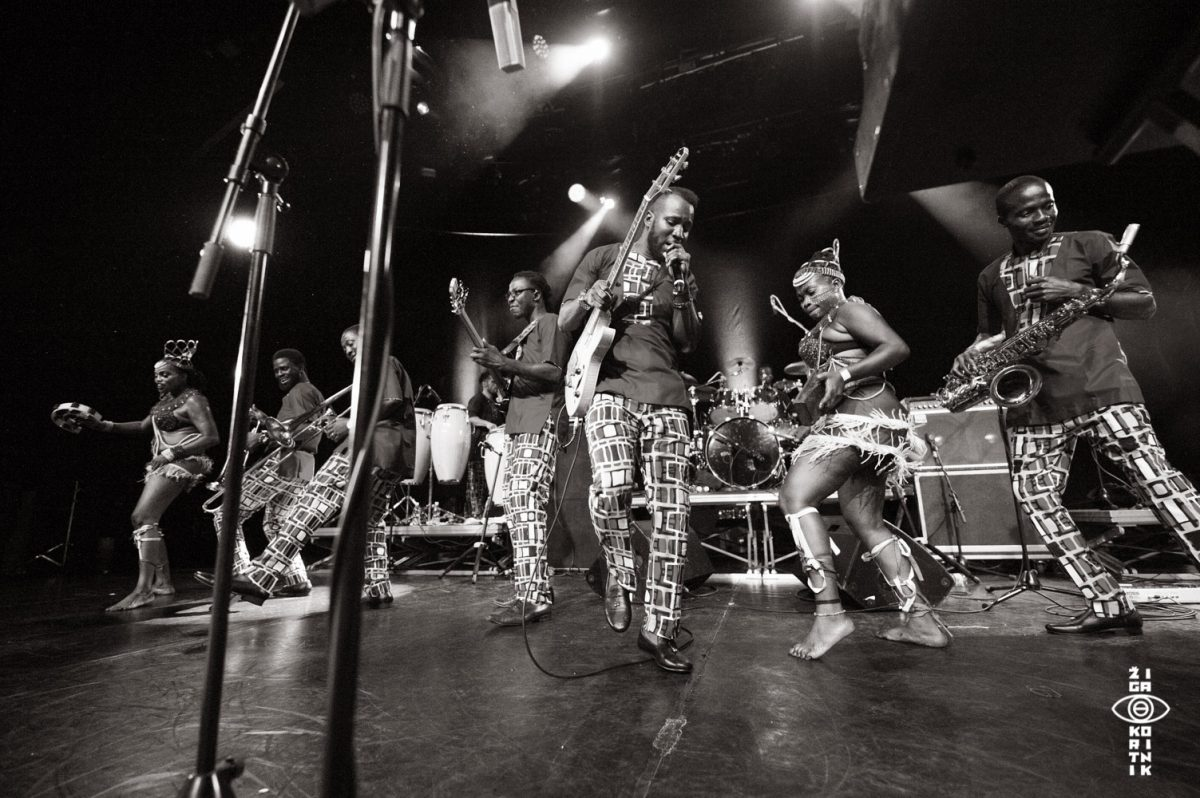Femi Kuti & Positive Force
•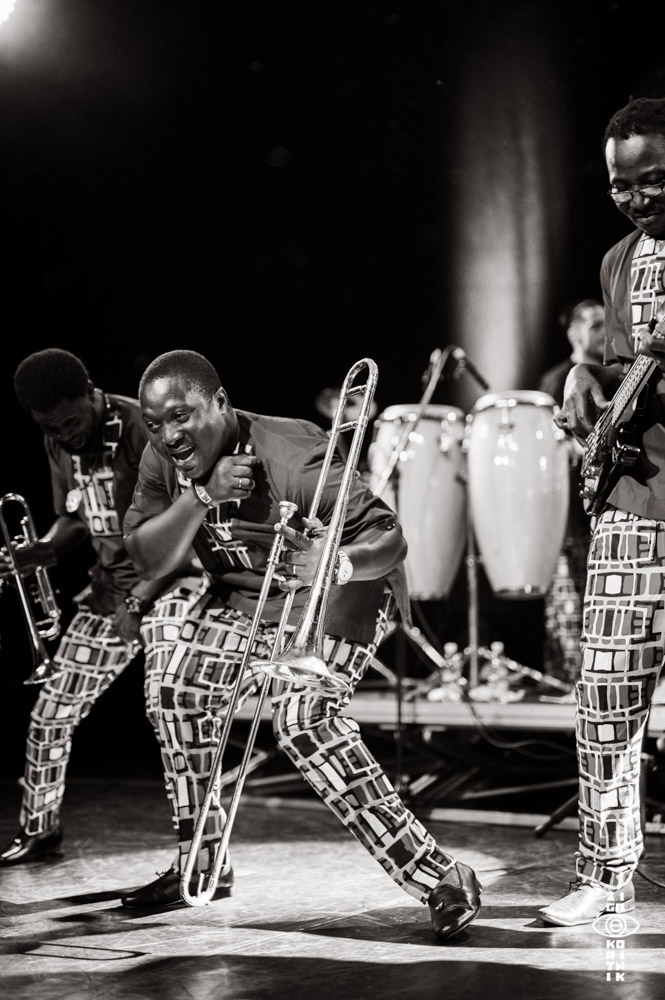Femi Kuti & Positive Force
•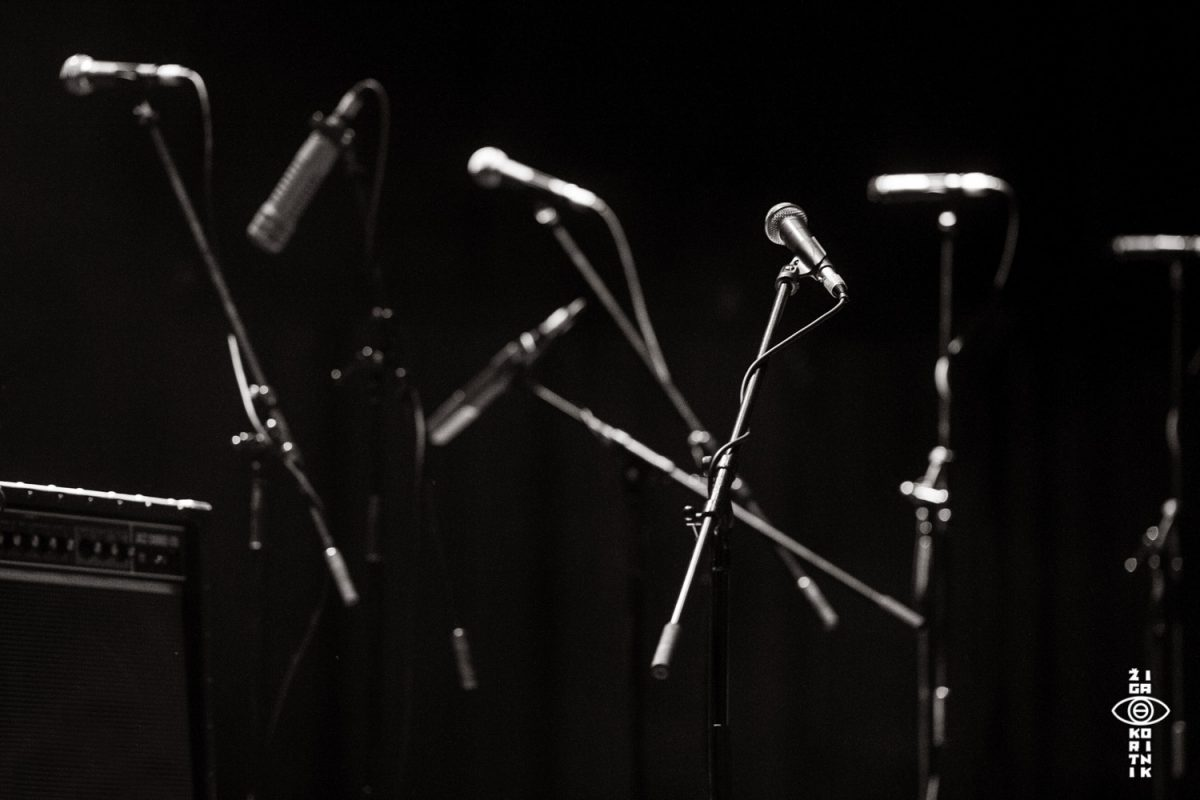FemiKuti_ByZigaKoritnik2018